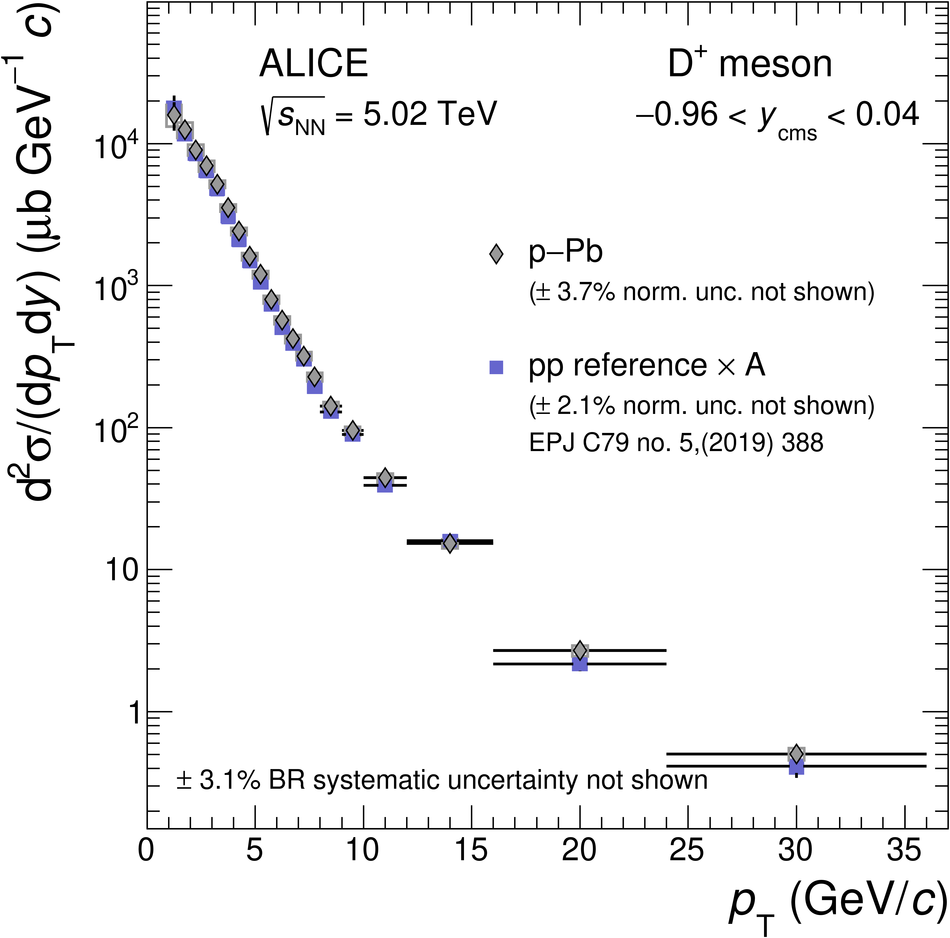# Figure 7

 $\pt$-differential production cross sections of prompt $\Dzero$, $\Dplus$, $\Dstar$, and $\Ds$ mesons with $-0.96< y_{\rm cms}< 0.04$ in p--Pb collisions at $\sqrtsNN=5.02 \tev$ compared with the respective pp reference cross sections  scaled by the Pb mass number ($A=208$) and corrected for the rapidity shift. For the $\Dzero$ meson, the results in the range $0< \pt< 1 \gev/c$ are obtained from the analysis that was performed without decay-vertex reconstruction, while those in the range \mbox{$1< \pt< 36 \gev/c$} are taken from the analysis with decay-vertex reconstruction The vertical bars and the empty boxes represent the statistical and systematic uncertainties